1. 清华大学 机械工程系, 北京 100084, 中国;
2. 浙江清华长三角研究院, 嘉兴 314006, 中国;
3. 福冈工业大学 智能机械工程系, 福冈 811-0295, 日本

Experimental study of the T(0, 1)-wave excitation method based on a buffer waveguide
SUN Feiran1, DING Yulin1, SUN Zhenguo1,2, CHEN Qiang1,2, MURAYAMA Riichi3
1. Department of Mechanical Engineering, Tsinghua University, Beijing 100084, China;
2. Yangtze Delta Region Institute of Tsinghua University, Jiaxing 314006, China;
3. Department of Intelligent Mechanical Engineering, Fukuoka Institute of Technology, Fukuoka 811-0295, Japan
Abstract: This paper presents an experimental study of the T(0, 1) mode guided wave excitation method for a buffer waveguide wrapped around a pipe which has been proposed to overcome the main challenge in online inspection of high-temperature pipelines. The dispersion curve was analyzed in tests to show that the T(0, 1) mode guided wave can be excited in the pipe by exciting the S0 mode Lamb wave in the buffer waveguide with a meander-line coil EMAT to generate the S0-wave and a PPM EMAT to detect the T(0, 1)-wave. Tests indicate that the T(0, 1)-wave can be converted from the S0-wave by the buffer waveguide, which verifies the validity of this method as a foundation for engineering applications.
Key words: guided wave     buffer waveguide     T (0, 1) mode     pipe inspection     electromagnetic acoustic transducer (EMAT)     high-temperature pipe图 1 基于缓冲波导的高温管道T(0, 1)模态导波激励方法

1 缓冲波导的参数设计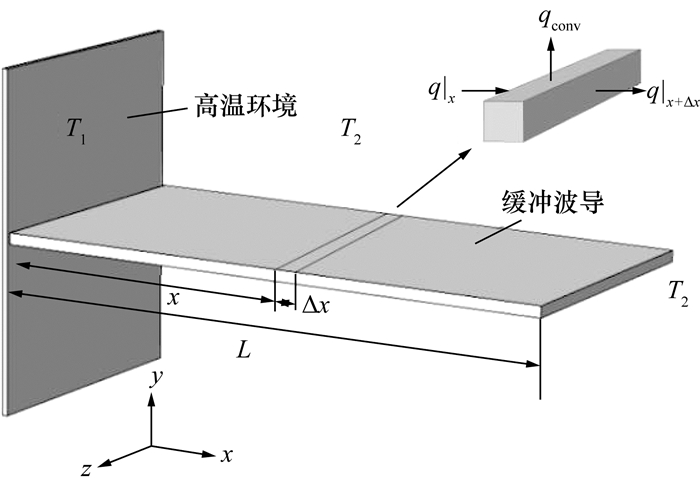图 2 缓冲波导温度分布的计算模型

 $q\left| {_x} \right. = q\left| {_{x + \Delta x}} \right. + {q_{{\rm{conv}}}},$ (1)
 $\left\{ \begin{array}{l} q\left| {_x} \right. = - mA\frac{{{\rm{d}}T}}{{{\rm{d}}x}}\left| {_x} \right.,\\ q\left| {_{x + \Delta x}} \right. = - mA\frac{{{\rm{d}}T}}{{{\rm{d}}x}}\left| {_{x + \Delta x}} \right.,\\ {q_{{\rm{conv}}}} = f \cdot \left( {P\Delta x} \right)\left( {T - {T_2}} \right). \end{array} \right.$ (2)

 $\frac{{{{\rm{d}}^2}T}}{{{\rm{d}}{x^2}}} - \frac{{fP}}{{mA}}\left( {T - {T_2}} \right) = 0.$ (3)

$n = {\left( {fP/mA} \right)^{1/2}}, {\rm{ }}\theta = T - {T_2}$, 可得通解的表达式：

 $\theta \left( x \right) = {C_1}{{\rm{e}}^{nx}} + {C_2}{{\rm{e}}^{ - nx}}.$ (4)

 $\left\{ \begin{array}{l} \theta \left( 0 \right) = {C_1} + {C_2} = {T_1} - {T_2},\\ - m\frac{{{\rm{d}}\theta }}{{{\rm{d}}x}}\left| {_L} \right. = f\theta \left( L \right). \end{array} \right.$ (5)

 $\frac{{\theta \left( x \right)}}{{\theta \left( 0 \right)}} = \frac{{\cosh \left[ {n\left( {L - x} \right)} \right] - \frac{f}{{nm}}\sinh \left[ {n\left( {L - x} \right)} \right]}}{{\cosh nL - \frac{f}{nm}\sinh nL}}.$ (6)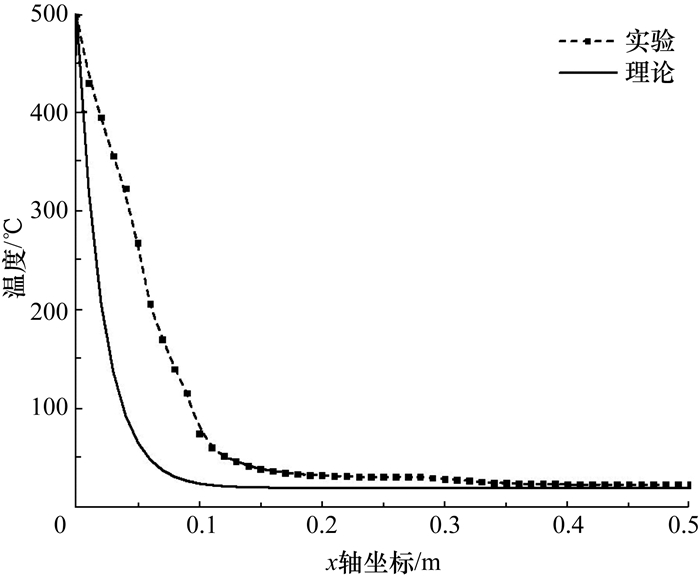图 3 缓冲波导内的温度分布曲线

2 超声导波频散曲线的建立

Lamb波在平板中的传播分为对称模态和反对称模态2种模态，其频散方程分别如式(7)和(8)所示

 $\frac{{\tan \left( {qh} \right)}}{{\tan \left( {ph} \right)}} = - \frac{{4{k^2}pq}}{{{{\left( {{q^2} - {k^2}} \right)}^2}}},$ (7)
 $\frac{{\tan \left( {qh} \right)}}{{\tan \left( {ph} \right)}} = - \frac{{{{\left( {{q^2} - {k^2}} \right)}^2}}}{{4{k^2}pq}}.$ (8)

 ${p^2} = \frac{{{\omega ^2}}}{{C_{\rm{L}}^2}} - {k^2},$ (9)
 ${q^2} = \frac{{{\omega ^2}}}{{C_{\rm{T}}^2}} - {k^2}.$ (10)

 $\left| {{C_{ij}}} \right| = 0.$ (11)

 参数 管道 缓冲波导 管道外径(波导宽度)/mm 21.35 10 厚度/mm 3.5 0.6 横波波速/(m·s-1) 3 100 3 167 纵波波速/(m·s-1) 5 800 5 925 Young模量/GPa 196 205 Poisson比 0.3 0.3 密度/(kg·m-3) 7 860 7 840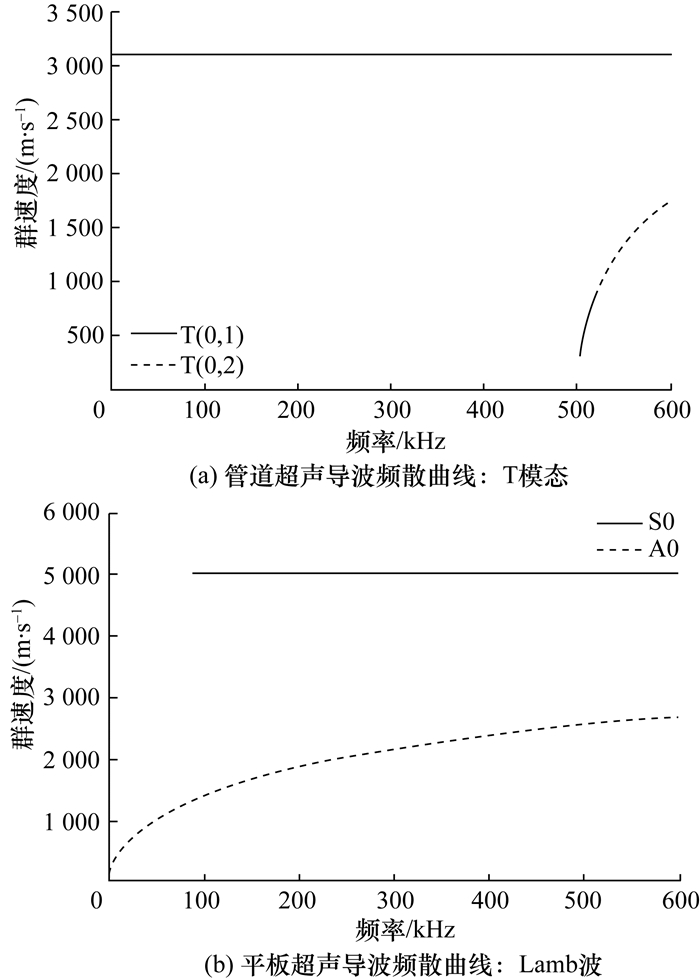图 4 超声导波的频散曲线

3 电磁超声换能器的设计和研制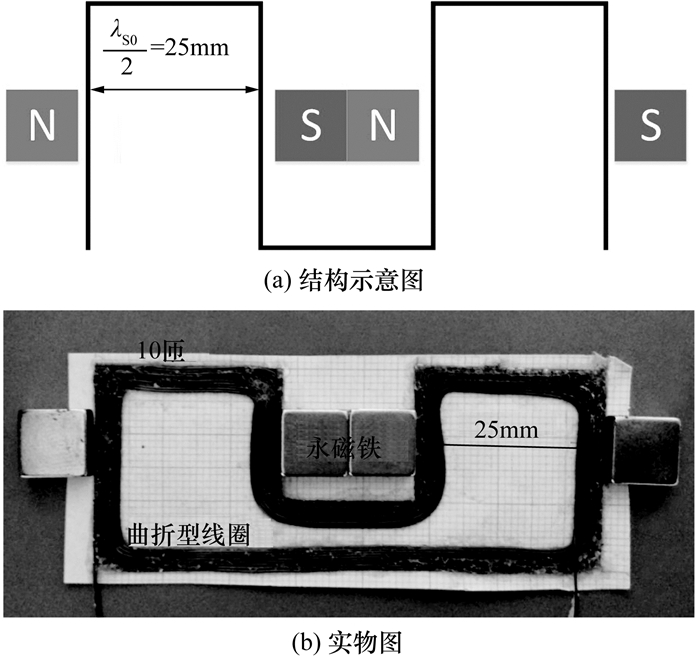图 5 曲折型线圈EMAT图 6 圆周式PPM EMAT

4 实验验证 4.1 实验装置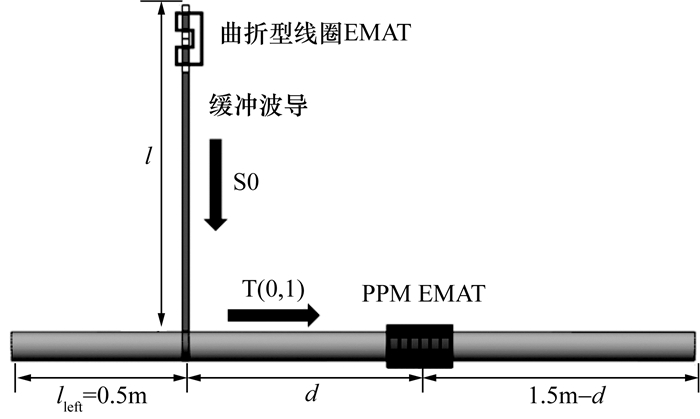图 7 基于缓冲波导激励T(0, 1)模态导波的实验装置

4.2 实验结果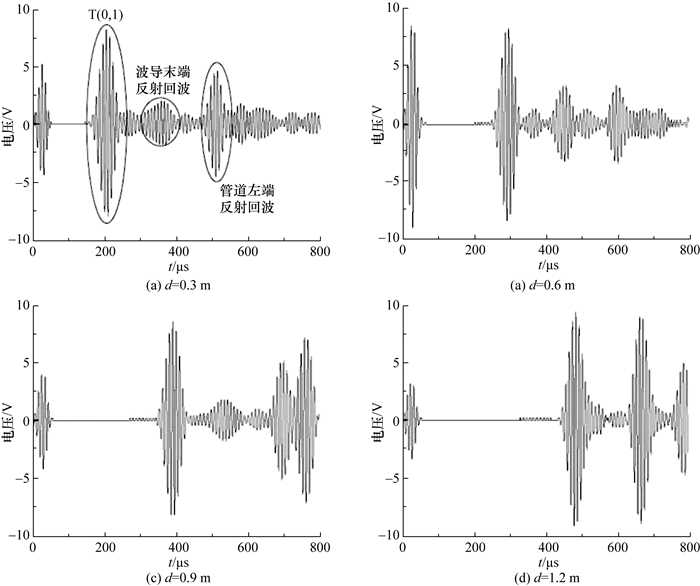图 8 S0模态导波通过缓冲波导后转换为T(0, 1)模态导波的波形图

 $\frac{l}{{{C_{{\rm{S0}}}}}} + \frac{d}{{{C_{{\rm{T}}\left( {0,1} \right)}}}} = \frac{{0.5{\rm{m}}}}{{5150{\rm{m/s}}}} + \frac{{0.3{\rm{m}}}}{{3100{\rm{m/s}}}} = 193.9\mu {\rm{s,}}$ (12)

 $\begin{array}{*{20}{c}} {\frac{l}{{{C_{{\rm{S0}}}}}} + \frac{d}{{{C_{{\rm{T}}\left( {0,1} \right)}}}} + \frac{{2l}}{{{C_{{\rm{S0}}}}}} = }\\ {\frac{{0.5{\rm{m}}}}{{5150{\rm{m/s}}}} + \frac{{0.3{\rm{m}}}}{{3100{\rm{m/s}}}} + \frac{{2 \times 0.5{\rm{m}}}}{{5150{\rm{m/s}}}} = 388.0\mu {\rm{s,}}} \end{array}$ (13)

 $\begin{array}{*{20}{c}} {\frac{l}{{{C_{{\rm{S0}}}}}} + \frac{d}{{{C_{{\rm{T}}\left( {0,1} \right)}}}} + \frac{{2 \times {l_{{\rm{left}}}}}}{{{C_{{\rm{T}}\left( {0,1} \right)}}}} = }\\ {\frac{{0.5{\rm{m}}}}{{5150{\rm{m/s}}}} + \frac{{0.3{\rm{m}}}}{{3100{\rm{m/s}}}} + \frac{{2 \times 0.5{\rm{m}}}}{{5150{\rm{m/s}}}} = 516.4\mu {\rm{s}}.} \end{array}$ (14)

5 结论

  ZHANG X W, TANG Z F, LV F Z, et al. Helical comb magnetostrictive patch transducers for inspecting spiral welded pipes using flexural guided waves[J]. Ultrasonics, 2017, 74: 1-10.  LIU Z H, HU Y A, FAN J W, et al. Longitudinal mode magnetostrictive patch transducer array employing a multi-splitting meander coil for pipe inspection[J]. NDT & E International, 2016, 79: 30-37.  XU J, WU X J, KONG D Y, et al. A guided wave sensor based on the inverse magnetostrictive effect for distinguishing symmetric from asymmetric features in pipes[J]. Sensors, 2015, 15(3): 5151-5162. DOI:10.3390/s150305151  何存富, 胡跃刚, 焦敬品, 等. 低阶扭转模态电磁声阵列传感器研制及其在厚壁小径管中的试验研究[J]. 机械工程学报, 2015, 51(2): 14-20. HE C F, HU Y G, JIAO J P, et al. Array of fundamental torsional mode EMATs and experiment in thick-wall pipe with small diameter[J]. Journal of Mechanical Engineering, 2015, 51(2): 14-20. (in Chinese)  黄焕东. 高温压力管道检测技术研究[C]//压力管道技术研究进展精选集——第四届全国管道技术学术会议. 黄山, 中国: 化学工业出版社, 2010: 406-408. HUANG H D. Research on the inspection technology of high-temperature pressure pipeline[C]//Pressure Piping Technology Progress Collection-Fourth National Pipeline Technology Conference. Huangshan, China: Chemical Industry Press, 2010: 406-408. (in Chinese)  DHUTTI A, TUMIN S A, MOHIMI A, et al. Development of low frequency high temperature ultrasonic transducers for in-service monitoring of pipework in power plants[J]. Procedia Engineering, 2016, 168: 983-986.  HERNANDEZ-VALLE F, DIXON S. Preliminary tests to design an EMAT with pulsed electromagnet for high temperature[C]//AIP Conference Proceedings. Chicago, Illinois: AIP, 2009, 1096(1): 936-941.  HERNANDEZ-VALLE F, DIXON S. Initial tests for designing a high temperature EMAT with pulsed electromagnet[J]. NDT & E International, 2010, 43(2): 171-175.  MURAYAMA R, MATSUMOTO K, USHITANI K, et al. Pipe inspection system by guide wave using a long distance waveguide[J]. Modern Mechanical Engineering, 2015, 5(4): 139-149.  丁辉. 计算超声学:声场分析及应用[M]. 北京: 科学出版社, 2010. Ding H. Computational ultrasonics-analysis and application of ultrasonic field[M]. Beijing: Science Press, 2010. (in Chinese)